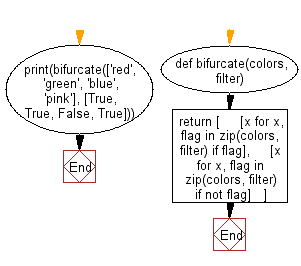﻿ Python: Split values into two groups, based on the result of the given filter list - w3resource# Python: Split values into two groups, based on the result of the given filter list

## Python List: Exercise - 231 with Solution

Write a Python program to split values into two groups, based on the result of the given filter list.

• Use a list comprehension and zip() to add elements to groups, based on filter.
• If filter has a truthy value for any element, add it to the first group, otherwise add it to the second group.

Sample Solution:

Python Code:

``````def bifurcate(colors, filter):
return [
[x for x, flag in zip(colors, filter) if flag],
[x for x, flag in zip(colors, filter) if not flag]
]
print(bifurcate(['red', 'green', 'blue', 'pink'], [True, True, False, True]))
```
```

Sample Output:

```[['red', 'green', 'pink'], ['blue']]
```

Flowchart:## Visualize Python code execution:

The following tool visualize what the computer is doing step-by-step as it executes the said program:

Python Code Editor:

Have another way to solve this solution? Contribute your code (and comments) through Disqus.

What is the difficulty level of this exercise?

Test your Python skills with w3resource's quiz

﻿

## Python: Tips of the Day

Floor Division:

When we speak of division we normally mean (/) float division operator, this will give a precise result in float format with decimals.

For a rounded integer result there is (//) floor division operator in Python. Floor division will only give integer results that are round numbers.

```print(1000 // 300)
print(1000 / 300)```

Output:

```3
3.3333333333333335```